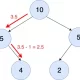###### Guidelines to use Transfer Learning in Convolutional Neural Networks
2022-09-20###### SumTree data structure for Prioritized Experience Replay (PER) explained with Python Code
2022-11-09

Scikit-learn, a popular Python library for machine learning, is often one of the first tools introduced to data science beginners. Its functions and methods cover the entire workflow of machine learning, including feature preprocessing and model evaluation, in addition to implementing machine learning algorithms.

This article focuses on a new API related to data preprocessing functions. In machine learning, features from raw data often require extensive preprocessing for optimal results. For example, some algorithms perform poorly if feature value ranges differ significantly, leading to biased results where features with higher values are given more weight.

For instance, in a house price prediction problem, the area of a house may be around 200 square meters, while the age is usually less than 20, and the number of bedrooms can be 1, 2, or 3. All these features play a critical role in determining a house’s price, but machine learning models may give more weight to features with higher values if they are not scaled appropriately. Models tend to perform better and converge faster when features are on a relatively similar scale.

To address this issue, Scikit-learn’s preprocessing module offers functions to scale features to similar ranges. However, these functions return a NumPy array instead of a DataFrame, which can make it challenging to keep track of feature names. Therefore, in most cases, additional lines of code are required in the script to maintain the feature names.

Let’s do an example on the famous iris dataset.

```from sklearn.datasets import load_iris
from sklearn.model_selection import train_test_split
X_train, X_test, y_train, y_test = train_test_split(
X, y, stratify=y, random_state=0
)
```

We can train a standard scaler on the train set and use it to transform the feature values in the test set.

```from sklearn.preprocessing import StandardScaler
scaler = StandardScaler()
scaler.fit(X_train)
X_test_scaled = scaler.transform(X_test)
type(X_test_scaled)
# output
numpy.ndarray
```

The variable X_test is a Pandas DataFrame whereas X_test_scaled is a NumPy array. It would be much more practical to also have X_test_scaled as a DataFrame.

It is time to share the good news now! ️

# The set_output API

The new set_output API allows for configuring transformers to output pandas DataFrames. It is still in an unstable release so I will share the example from the official documentation. The same example as we did above but with a small addition of the set_output.

```from sklearn.preprocessing import StandardScaler
scaler = StandardScaler().set_output(transform="pandas")
scaler.fit(X_train)
X_test_scaled = scaler.transform(X_test)